# Oct 2015

I will post seven new problems every weekend, one for each day of the week. The idea is that you work on your own during the week and I will post answers on the following Friday at 7pm on the morning of the following Saturday.

For instructions on submitting weekly answers, click on the “Logistics” tab.

For previously posted problems, click on the “Archive” tab.

Prizes:  As encouragement, JerseySTEM is offering prizes to recognize those who have consistently answered questions correctly. The first round of prizes will be given out on Friday, Oct 30 23, 2015, at Tech: Vineyard (232 Main Street, Chatham), to recognize high performers for the time period Sept 12 – Oct 16, 2015.

Week of Oct 24 – Oct 30: This week’s focus is on visualizing cubes.  Click GoogleForm to submit this week’s answers.

Answers: A, D, C, B, D, C, E

Oct 24: Six squares are colored, front and back, (R = red, B = blue, O = orange, Y = yellow, G = green, and W = white). They are hinged together as shown, then folded to form a cube. The face opposite the white face is$[asy] draw((0,2)--(1,2)--(1,1)--(2,1)--(2,0)--(3,0)--(3,1)--(4,1)--(4,2)--(2,2)--(2,3)--(0,3)--cycle); draw((1,3)--(1,2)--(2,2)--(2,1)--(3,1)--(3,2)); label($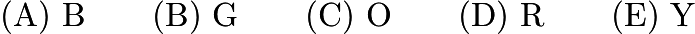$\text{(A)}\ \text{B} \qquad \text{(B)}\ \text{G} \qquad \text{(C)}\ \text{O} \qquad \text{(D)}\ \text{R} \qquad \text{(E)}\ \text{Y}$

Source: AMC8 1999

Oct 25: Three dice with faces numbered 1 through 6 are stacked as shown. Seven of the eighteen faces are visible, leaving eleven faces hidden (back, bottom, between). The total number of dots NOT visible in this view is$[asy] draw((0,0)--(2,0)--(3,1)--(3,7)--(1,7)--(0,6)--cycle); draw((3,7)--(2,6)--(0,6)); draw((3,5)--(2,4)--(0,4)); draw((3,3)--(2,2)--(0,2)); draw((2,0)--(2,6)); dot((1,1)); dot((.5,.5)); dot((1.5,.5)); dot((1.5,1.5)); dot((.5,1.5)); dot((2.5,1.5)); dot((.5,2.5)); dot((1.5,2.5)); dot((1.5,3.5)); dot((.5,3.5)); dot((2.25,2.75)); dot((2.5,3)); dot((2.75,3.25)); dot((2.25,3.75)); dot((2.5,4)); dot((2.75,4.25)); dot((.5,5.5)); dot((1.5,4.5)); dot((2.25,4.75)); dot((2.5,5.5)); dot((2.75,6.25)); dot((1.5,6.5)); [/asy]$$\text{(A)}\ 21 \qquad \text{(B)}\ 22 \qquad \text{(C)}\ 31 \qquad \text{(D)}\ 41 \qquad \text{(E)}\ 53$

Source: AMC8 2000

Oct 26: Six cubes, each an inch on an edge, are fastened together, as shown. Find the total surface area in square inches. Include the top, bottom and sides.$[asy]/* AMC8 2002 #22 Problem */ draw((0,0)--(0,1)--(1,1)--(1,0)--cycle); draw((0,1)--(0.5,1.5)--(1.5,1.5)--(1,1)); draw((1,0)--(1.5,0.5)--(1.5,1.5)); draw((0.5,1.5)--(1,2)--(1.5,2)); draw((1.5,1.5)--(1.5,3.5)--(2,4)--(3,4)--(2.5,3.5)--(2.5,0.5)--(1.5,.5)); draw((1.5,3.5)--(2.5,3.5)); draw((1.5,1.5)--(3.5,1.5)--(3.5,2.5)--(1.5,2.5)); draw((3,4)--(3,3)--(2.5,2.5)); draw((3,3)--(4,3)--(4,2)--(3.5,1.5)); draw((4,3)--(3.5,2.5)); draw((2.5,.5)--(3,1)--(3,1.5));[/asy]$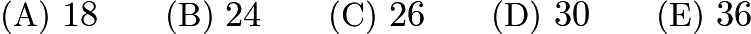$\text{(A)}\ 18 \qquad \text{(B)}\ 24 \qquad \text{(C)}\ 26 \qquad \text{(D)}\ 30 \qquad \text{(E)}\ 36$

Source: AMC8 2002

Oct 27: Fourteen white cubes are put together to form the figure on the right. The complete surface of the figure, including the bottom, is painted red. The figure is then separated into individual cubes. How many of the individual cubes have exactly four red faces?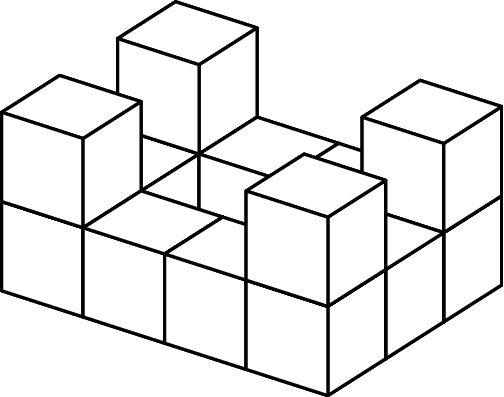$[asy] import three; defaultpen(linewidth(0.8)); real r=0.5; currentprojection=orthographic(3/4,8/15,7/15); draw(unitcube, white, thick(), nolight); draw(shift(1,0,0)*unitcube, white, thick(), nolight); draw(shift(2,0,0)*unitcube, white, thick(), nolight); draw(shift(0,0,1)*unitcube, white, thick(), nolight); draw(shift(2,0,1)*unitcube, white, thick(), nolight); draw(shift(0,1,0)*unitcube, white, thick(), nolight); draw(shift(2,1,0)*unitcube, white, thick(), nolight); draw(shift(0,2,0)*unitcube, white, thick(), nolight); draw(shift(2,2,0)*unitcube, white, thick(), nolight); draw(shift(0,3,0)*unitcube, white, thick(), nolight); draw(shift(0,3,1)*unitcube, white, thick(), nolight); draw(shift(1,3,0)*unitcube, white, thick(), nolight); draw(shift(2,3,0)*unitcube, white, thick(), nolight); draw(shift(2,3,1)*unitcube, white, thick(), nolight);[/asy]$$\textbf{(A)}\ 4\qquad\textbf{(B)}\ 6\qquad\textbf{(C)}\ 8\qquad\textbf{(D)}\ 10\qquad\textbf{(E)}\ 12$

Source: AMC8 2003

Oct 28: A shape is created by joining seven unit cubes, as shown. What is the ratio of the volume in cubic units to the surface area in square units?$[asy] import three; defaultpen(linewidth(0.8)); real r=0.5; currentprojection=orthographic(1,1/2,1/4); draw(unitcube, white, thick(), nolight); draw(shift(1,0,0)*unitcube, white, thick(), nolight); draw(shift(1,-1,0)*unitcube, white, thick(), nolight); draw(shift(1,0,-1)*unitcube, white, thick(), nolight); draw(shift(2,0,0)*unitcube, white, thick(), nolight); draw(shift(1,1,0)*unitcube, white, thick(), nolight); draw(shift(1,0,1)*unitcube, white, thick(), nolight);[/asy]$$\textbf{(A)} \:1 : 6 \qquad\textbf{ (B)}\: 7 : 36 \qquad\textbf{(C)}\: 1 : 5 \qquad\textbf{(D)}\: 7 : 30\qquad\textbf{ (E)}\: 6 : 25$

Source: AMC8 2008

Oct 29: A cube has edge length 2. Suppose that we glue a cube of edge length 1 on top of the big cube so that one of its faces rests entirely on the top face of the larger cube. The percent increase in the surface area (sides, top, and bottom) from the original cube to the new solid formed is closest to$[asy] draw((0,0)--(2,0)--(3,1)--(3,3)--(2,2)--(0,2)--cycle); draw((2,0)--(2,2)); draw((0,2)--(1,3)); draw((1,7/3)--(1,10/3)--(2,10/3)--(2,7/3)--cycle); draw((2,7/3)--(5/2,17/6)--(5/2,23/6)--(3/2,23/6)--(1,10/3)); draw((2,10/3)--(5/2,23/6)); draw((3,3)--(5/2,3)); [/asy]$$\text{(A)}\ 10 \qquad \text{(B)}\ 15 \qquad \text{(C)}\ 17 \qquad \text{(D)}\ 21 \qquad \text{(E)}\ 25$

Source: AMC8 2000

Oct 30: A one-cubic-foot cube is cut into four pieces by three cuts parallel to the top face of the cube. The first cut is$1/2$foot from the top face. The second cut is$1/3$ foot below the first cut, and the third cut is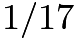$1/17$ foot below the second cut. From the top to the bottom the pieces are labeled A, B, C, and D. The pieces are then glued together end to end as shown in the second diagram. What is the total surface area of this solid in square feet?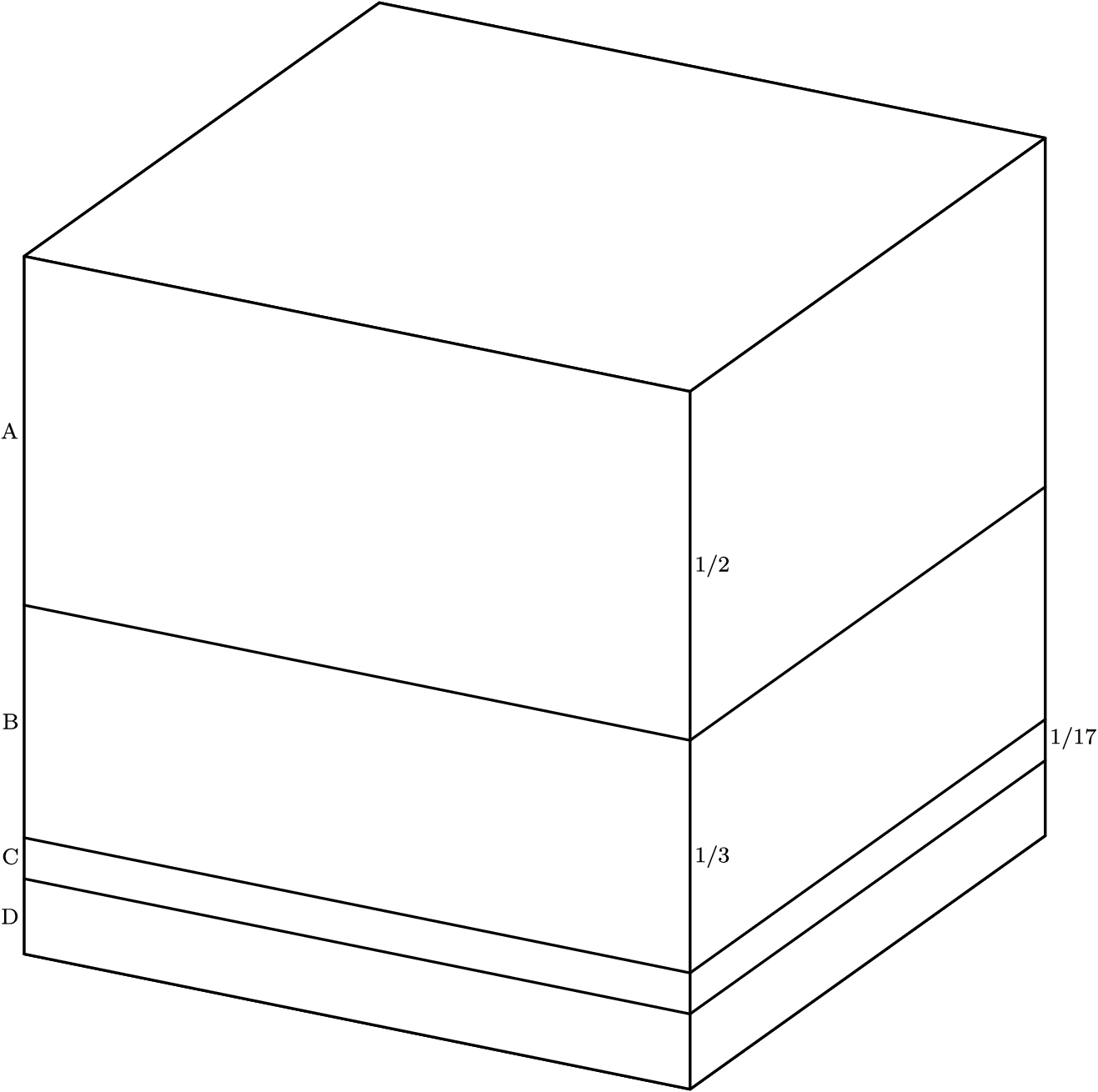$[asy] import three; real d=11/102; defaultpen(fontsize(8)); defaultpen(linewidth(0.8)); currentprojection=orthographic(1,8/15,7/15); draw(unitcube, white, thick(), nolight); void f(real x) { draw((0,1,x)--(1,1,x)--(1,0,x)); } f(d); f(1/6); f(1/2); label($$[asy] import three; real d=11/102; defaultpen(fontsize(8)); defaultpen(linewidth(0.8)); currentprojection=orthographic(2,8/15,7/15); int t=0; void f(real x) { path3 r=(t,1,x)--(t+1,1,x)--(t+1,1,0)--(t,1,0)--cycle; path3 f=(t+1,1,x)--(t+1,1,0)--(t+1,0,0)--(t+1,0,x)--cycle; path3 u=(t,1,x)--(t+1,1,x)--(t+1,0,x)--(t,0,x)--cycle; draw(surface(r), white, nolight); draw(surface(f), white, nolight); draw(surface(u), white, nolight); draw((t,1,x)--(t+1,1,x)--(t+1,1,0)--(t,1,0)--(t,1,x)--(t,0,x)--(t+1,0,x)--(t+1,1,x)--(t+1,1,0)--(t+1,0,0)--(t+1,0,x)); t=t+1; } f(d); f(1/2); f(1/3); f(1/17); label($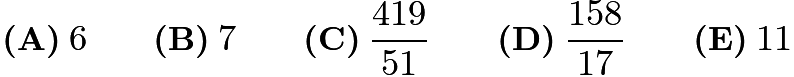$\textbf{(A)}\:6\qquad\textbf{(B)}\:7\qquad\textbf{(C)}\:\frac{419}{51}\qquad\textbf{(D)}\:\frac{158}{17}\qquad\textbf{(E)}\:11$

Source: AMC8 2009

Week of Oct. 17 – Oct. 23: This week’s focus is on interpreting graphs.  Note that this week’s problems count towards the next prize period.  So everybody has a fresh start!  Also starting this week I will post answers and new problems on Sat mornings, instead of Fri at 7pm.   This will give those of you who are last-minute people Fri night to submit the answers :).  Click GoogleForm to submit this week’s answers.

Answers: D, E, E, A, A, D, B

Oct 17: Candy sales from the Boosters Club from January through April are shown. What were the average sales per month in dollars?$[asy] draw((0,0)--(36,0)--(36,24)--(0,24)--cycle); draw((0,4)--(36,4)); draw((0,8)--(36,8)); draw((0,12)--(36,12)); draw((0,16)--(36,16)); draw((0,20)--(36,20)); fill((4,0)--(8,0)--(8,20)--(4,20)--cycle, black); fill((12,0)--(16,0)--(16,12)--(12,12)--cycle, black); fill((20,0)--(24,0)--(24,8)--(20,8)--cycle, black); fill((28,0)--(32,0)--(32,24)--(28,24)--cycle, black); label($$\textbf{(A)}\ 60\qquad\textbf{(B)}\ 70\qquad\textbf{(C)}\ 75\qquad\textbf{(D)}\ 80\qquad\textbf{(E)}\ 85$

Source: AMC8 2008

Oct 18:$650$ students were surveyed about their pasta preferences. The choices were lasagna, manicotti, ravioli and spaghetti. The results of the survey are displayed in the bar graph. What is the ratio of the number of students who preferred spaghetti to the number of students who preferred manicotti?$\mathrm{(A)} \frac{2}{5} \qquad \mathrm{(B)} \frac{1}{2} \qquad \mathrm{(C)} \frac{5}{4} \qquad \mathrm{(D)} \frac{5}{3} \qquad \mathrm{(E)} \frac{5}{2}$

Source: AMC8 2007

Oct 19: The results of a cross-country team’s training run are graphed below. Which student has the greatest average speed?$[asy] for ( int i = 1; i <= 7; ++i ) { draw((i,0)--(i,6)); } for ( int i = 1; i <= 5; ++i ) { draw((0,i)--(8,i)); } draw((-0.5,0)--(8,0), linewidth(1)); draw((0,-0.5)--(0,6), linewidth(1)); label($$\textbf{(A)}\ \text{Angela}\qquad\textbf{(B)}\ \text{Briana}\qquad\textbf{(C)}\ \text{Carla}\qquad\textbf{(D)}\ \text{Debra}\qquad\textbf{(E)}\ \text{Evelyn}$

Source: AMC8 2005

Oct 20: Jorge’s teacher asks him to plot all the ordered pairs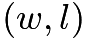$(w, l)$ of positive integers for which$w$ is the width and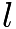$l$ is the length of a rectangle with area 12. What should his graph look like?$\textbf{(A)}$$[asy] size(75); draw((0,-1)--(0,13)); draw((-1,0)--(13,0)); dot((1,12)); dot((2,6)); dot((3,4)); dot((4,3)); dot((6,2)); dot((12,1)); label($$\textbf{(B)}$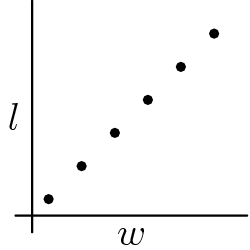$[asy] size(75); draw((0,-1)--(0,13)); draw((-1,0)--(13,0)); dot((1,1)); dot((3,3)); dot((5,5)); dot((7,7)); dot((9,9)); dot((11,11)); label($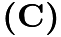$\textbf{(C)}$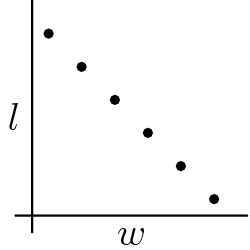$[asy] size(75); draw((0,-1)--(0,13)); draw((-1,0)--(13,0)); dot((1,11)); dot((3,9)); dot((5,7)); dot((7,5)); dot((9,3)); dot((11,1)); label($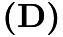$\textbf{(D)}$$[asy] size(75); draw((0,-1)--(0,13)); draw((-1,0)--(13,0)); dot((1,6)); dot((3,6)); dot((5,6)); dot((7,6)); dot((9,6)); dot((11,6)); label($$\textbf{(E)}$$[asy] size(75); draw((0,-1)--(0,13)); draw((-1,0)--(13,0)); dot((6,1)); dot((6,3)); dot((6,5)); dot((6,7)); dot((6,9)); dot((6,11)); label($

Source: AMC8 2006

Oct 21: Amanda draws five circles with radii$1, 2, 3, 4$ and$5$. Then for each circle she plots the point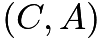$(C,A)$, where$C$ is its circumference and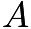$A$ is its area. Which of the following could be her graph?

Oct 22: Tess runs counterclockwise around rectangular block$JKLM$. She lives at corner$J$. Which graph could represent her straight-line distance from home?$[asy] unitsize(5mm); pair J=(-3,2); pair K=(-3,-2); pair L=(3,-2); pair M=(3,2); draw(J--K--L--M--cycle); label($Source: AMC8 2004

Oct 23: A ship travels from point$A$ to point$B$ along a semicircular path, centered at Island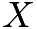$X$. Then it travels along a straight path from$B$ to$C$. Which of these graphs best shows the ship’s distance from Island$X$ as it moves along its course?$[asy]size(150); pair X=origin, A=(-5,0), B=(5,0), C=(0,5); draw(Arc(X, 5, 180, 360)^^B--C); dot(X); label($Source: AMC8 2003

Week of Oct. 10 – Oct. 16: This week’s focus is probability. Most of the seven problems appeared at the end of the tests, and so this is the hardest week yet.  Good luck! Click GoogleForm to submit this week’s answers.

Answers: B, E, B, D, B, E, B

Oct. 10: A board game spinner is divided into three regions labeled$A$,$B$ and$C$. The probability of the arrow stopping on region$A$ is$\frac{1}{3}$ and on region$B$ is$\frac{1}{2}$. The probability of the arrow stopping on region$C$ is$\text{(A)}\ \frac{1}{12} \qquad \text{(B)}\ \frac{1}{6} \qquad \text{(C)}\ \frac{1}{5} \qquad \text{(D)}\ \frac{1}{3} \qquad \text{(E)}\ \frac{2}{5}$

Source: AMC8 2002

Oct. 11: When a fair six-sided die is tossed on a table top, the bottom face cannot be seen. What is the probability that the product of the numbers on the five faces that can be seen is divisible by 6?$\textbf{(A)}\ \frac{1}{3}\qquad\textbf{(B)}\ \frac{1}{2}\qquad\textbf{(C)}\ \frac{2}{3}\qquad\textbf{(D)}\ \frac{5}{6}\qquad\textbf{(E)}\ 1$

Source: AMC8 2003

Oct. 12: Jeff rotates spinners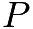$P$,$Q$ and$R$ and adds the resulting numbers. What is the probability that his sum is an odd number?$[asy] size(200); path circle=circle((0,0),2); path r=(0,0)--(0,2); draw(circle,linewidth(1)); draw(shift(5,0)*circle,linewidth(1)); draw(shift(10,0)*circle,linewidth(1)); draw(r,linewidth(1)); draw(rotate(120)*r,linewidth(1)); draw(rotate(240)*r,linewidth(1)); draw(shift(5,0)*r,linewidth(1)); draw(shift(5,0)*rotate(90)*r,linewidth(1)); draw(shift(5,0)*rotate(180)*r,linewidth(1)); draw(shift(5,0)*rotate(270)*r,linewidth(1)); draw(shift(10,0)*r,linewidth(1)); draw(shift(10,0)*rotate(60)*r,linewidth(1)); draw(shift(10,0)*rotate(120)*r,linewidth(1)); draw(shift(10,0)*rotate(180)*r,linewidth(1)); draw(shift(10,0)*rotate(240)*r,linewidth(1)); draw(shift(10,0)*rotate(300)*r,linewidth(1)); label($$\textbf{(A)}\ \frac{1}{4}\qquad\textbf{(B)}\ \frac{1}{3}\qquad\textbf{(C)}\ \frac{1}{2}\qquad\textbf{(D)}\ \frac{2}{3}\qquad\textbf{(E)}\ \frac{3}{4}$

Source: AMC8 2006

Oct. 13: Spinners$A$ and$B$ are spun. On each spinner, the arrow is equally likely to land on each number. What is the probability that the product of the two spinners’ numbers is even?$[asy] pair A=(0,0); pair B=(3,0); draw(Circle(A,1)); draw(Circle(B,1)); draw((-1,0)--(1,0)); draw((0,1)--(0,-1)); draw((3,0)--(3,1)); draw((3+sqrt(3)/2,-.5)--(3,0)); draw((3,0)--(3-sqrt(3)/2,-.5)); label($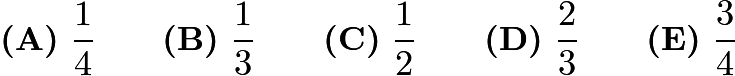$\textbf{(A)}\ \frac14\qquad \textbf{(B)}\ \frac13\qquad \textbf{(C)}\ \frac12\qquad \textbf{(D)}\ \frac23\qquad \textbf{(E)}\ \frac34$

Source: AMC8 2004

Oct. 14: At a party there are only single women and married men with their wives. The probability that a randomly selected woman is single is$\frac25$. What fraction of the people in the room are married men?$\textbf{(A)}\ \frac13\qquad \textbf{(B)}\ \frac38\qquad \textbf{(C)}\ \frac25\qquad \textbf{(D)}\ \frac{5}{12}\qquad \textbf{(E)}\ \frac35$

Source: AMC8 2004

Oct. 15: Harold tosses a nickel four times. The probability that he gets at least as many heads as tails is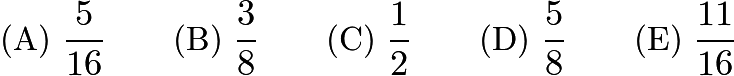$\text{(A)}\ \frac{5}{16} \qquad \text{(B)}\ \frac{3}{8} \qquad \text{(C)}\ \frac{1}{2} \qquad \text{(D)}\ \frac{5}{8} \qquad \text{(E)}\ \frac{11}{16}$

Source: AMC8 2002

Oct. 16: Keiko tosses one penny and Ephraim tosses two pennies. The probability that Ephraim gets the same number of heads that Keiko gets is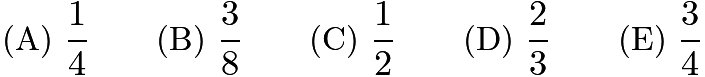$\text{(A)}\ \dfrac{1}{4} \qquad \text{(B)}\ \dfrac{3}{8} \qquad \text{(C)}\ \dfrac{1}{2} \qquad \text{(D)}\ \dfrac{2}{3} \qquad \text{(E)}\ \dfrac{3}{4}$

Source: AMC8 2000

Week of Oct. 3 – Oct. 9: This week’s 7 problems are about counting.  Next week’s will be on probability.  It is possible that counting and probability are harder than what I’ve posted in the past.  So don’t be discouraged if you find them a little bit more challenging :). Click GoogleForm to submit this week’s answers.

Answers: B, B, C, C, D, C, D

Oct 3: How many different four-digit numbers can be formed by rearranging the four digits in$2004$?$\textbf{(A)}\ 4\qquad\textbf{(B)}\ 6\qquad\textbf{(C)}\ 16\qquad\textbf{(D)}\ 24\qquad\textbf{(E)}\ 81$

Source: AMC8 2004

Oct 4: Find the number of two-digit positive integers whose digits total$7$.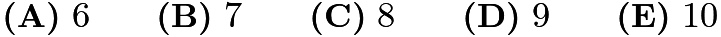$\textbf{(A)}\ 6 \qquad\textbf{(B)}\ 7 \qquad\textbf{(C)}\ 8 \qquad\textbf{(D)}\ 9 \qquad\textbf{(E)}\ 10$

Source: AMC8 2004

Oct 5: The number 64 has the property that it is divisible by its units digit. How many whole numbers between 10 and 50 have this property?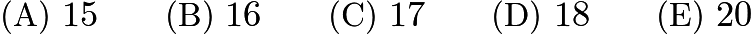$\text{(A)}\ 15 \qquad \text{(B)}\ 16 \qquad \text{(C)}\ 17 \qquad \text{(D)}\ 18 \qquad \text{(E)}\ 20$

Source: AMC8 2000

Oct 6: How many two-digit numbers have digits whose sum is a perfect square?$\textbf{(A)}\ 13\qquad\textbf{(B)}\ 16\qquad\textbf{(C)}\ 17\qquad\textbf{(D)}\ 18\qquad\textbf{(E)}\ 19$

Source: AMC8 2006

Oct 7: Bicycle license plates in Flatville each contain three letters. The first is chosen from the set {C,H,L,P,R}, the second from {A,I,O}, and the third from {D,M,N,T}.

When Flatville needed more license plates, they added two new letters. The new letters may both be added to one set or one letter may be added to one set and one to another set. What is the largest possible number of ADDITIONAL license plates that can be made by adding two letters?$\text{(A)}\ 24 \qquad \text{(B)}\ 30 \qquad \text{(C)}\ 36 \qquad \text{(D)}\ 40 \qquad \text{(E)}\ 60$

Source: AMC8 1999

Oct 8: How many three-digit numbers are divisible by 13?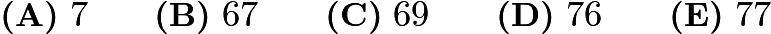$\textbf{(A)}\ 7\qquad\textbf{(B)}\ 67\qquad\textbf{(C)}\ 69\qquad\textbf{(D)}\ 76\qquad\textbf{(E)}\ 77$

Source: AMC8 2005

Oct 9: How many whole numbers between 99 and 999 contain exactly one 0?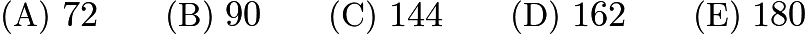$\text{(A)}\ 72 \qquad \text{(B)}\ 90 \qquad \text{(C)}\ 144 \qquad \text{(D)}\ 162 \qquad \text{(E)}\ 180$

Source: AMC8 2002